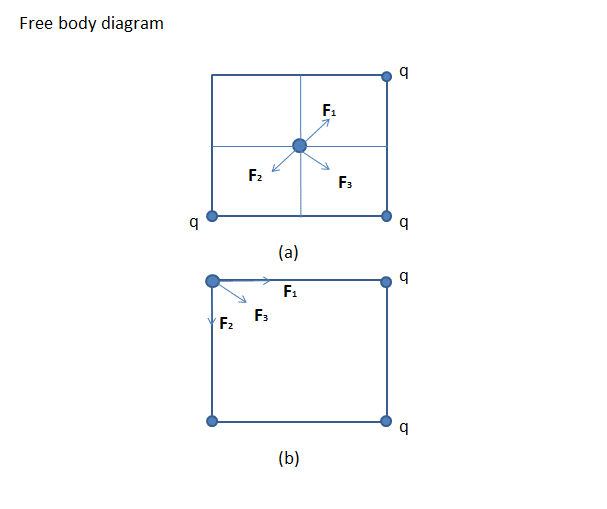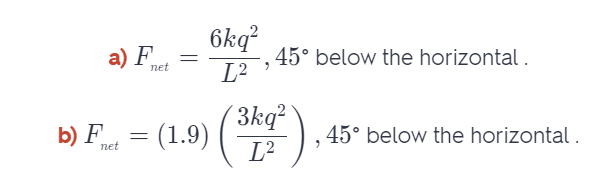# Three Identical Point Charges Q Are Placed At Each Of Three Corners Of A Square Of Side L.

We thoroughly check each answer to a question to provide you with the most correct answers. Found a mistake? Let us know about it through the REPORT button at the bottom of the page.

Three identical point charges q are placed at each of three corners of a square of side L. Find the magnitude and direction of the net force on a point charge -3q placed (a) at the center of the square and (b) at the vacant corner of the square.

In each case, draw a free-body diagram showing the forces exerted on the -3q charge by each of the other three charges.